Main Content

# GNSS Simulation Overview

Global Navigation Satellite System (GNSS) simulation generates receiver position estimates. These receiver position estimates come from GPS and GNSS sensor models as `gpsSensor` and `gnssSensor` objects. Monitor the status of the position estimate in the `gnssSensor` using the dilution of precision outputs and compare the number of satellites available.

### Simulation Parameters

Specify the parameters to be used in the GNSS simulation:

• Sampling rate of the GNSS receiver

• Local navigation reference frame

• Location on Earth in latitude, longitude, and altitude (LLA) coordinates

• Number of samples to simulate

```Fs = 1; refFrame = "NED"; lla0 = [42.2825 -71.343 53.0352]; N = 100;```

Create a trajectory for a stationary sensor.

```pos = zeros(N, 3); vel = zeros(N, 3); time = (0:N-1) ./ Fs;```

### Sensor Model Creation

Create the GNSS simulation objects, `gpsSensor` and `gnssSensor` using the same initial parameters for each.

```gps = gpsSensor("SampleRate", Fs, "ReferenceLocation", lla0, ... "ReferenceFrame", refFrame); gnss = gnssSensor("SampleRate", Fs, "ReferenceLocation", lla0, ... "ReferenceFrame", refFrame);```

### Simulation using `gpsSensor`

Generate outputs from a stationary receiver using the GPS sensor. Visualize the position in LLA coordinates and the velocity in each direction.

```% Generate outputs. [llaGPS, velGPS] = gps(pos, vel); % Visualize positon. figure subplot(3, 1, 1) plot(time, llaGPS(:,1)) title('Latitude') ylabel('degrees') xlabel('s') subplot(3, 1, 2) plot(time, llaGPS(:,2)) title('Longitude') ylabel('degrees') xlabel('s') subplot(3, 1, 3) plot(time, llaGPS(:,3)) title('Altitude') ylabel('m') xlabel('s')```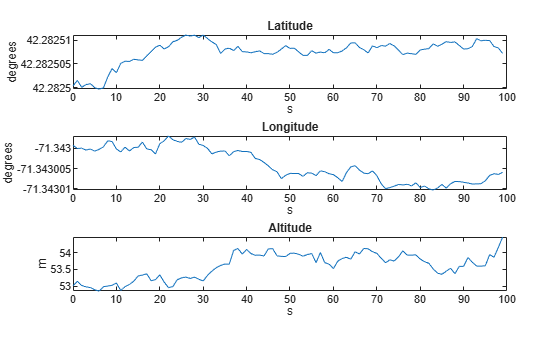```% Visualize velocity. figure plot(time, velGPS) title('Velocity') legend('X', 'Y', 'Z') ylabel('m/s') xlabel('s')```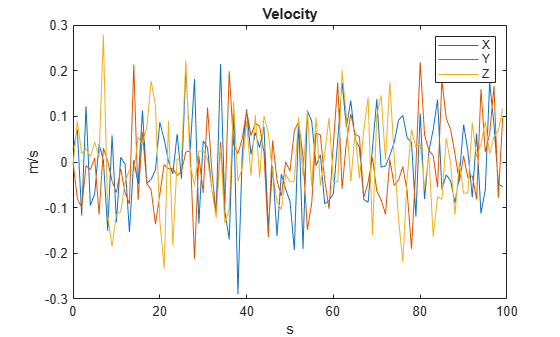### Simulation using `gnssSensor`

Generate outputs from a stationary receiver using the GNSS sensor. Visualize the position and velocity and notice the differences in the simulation.

```% Generate outputs. [llaGNSS, velGNSS] = gnss(pos, vel); % Visualize positon. figure subplot(3, 1, 1) plot(time, llaGNSS(:,1)) title('Latitude') ylabel('degrees') xlabel('s') subplot(3, 1, 2) plot(time, llaGNSS(:,2)) title('Longitude') ylabel('degrees') xlabel('s') subplot(3, 1, 3) plot(time, llaGNSS(:,3)) title('Altitude') ylabel('m') xlabel('s')```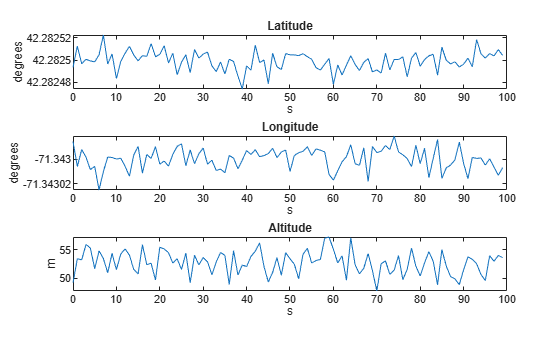```% Visualize velocity. figure plot(time, velGNSS) title('Velocity') legend('X', 'Y', 'Z') ylabel('m/s') xlabel('s')```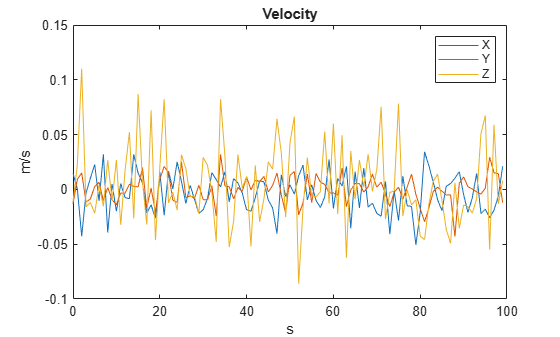### Dilution of Precision

The `gnssSensor` object has a higher fidelity simulation compared to `gpsSensor`. For example, the `gnssSensor` object uses simulated satellite positions to estimate the receiver position. This means that the horizontal dilution of precision (HDOP) and vertical dilution of precision (VDOP) can be reported along with the position estimate. These values indicate how precise the position estimate is based on the satellite geometry. Smaller values indicate a more precise estimate.

```% Set the RNG seed to reproduce results. rng('default') % Specify the start time of the simulation. initTime = datetime(2020, 4, 20, 18, 10, 0, "TimeZone", "America/New_York"); % Create the GNSS receiver model. gnss = gnssSensor("SampleRate", Fs, "ReferenceLocation", lla0, ... "ReferenceFrame", refFrame, "InitialTime", initTime); % Obtain the receiver status. [~, ~, status] = gnss(pos, vel); disp(status(1))```
``` SatelliteAzimuth: [7x1 double] SatelliteElevation: [7x1 double] HDOP: 1.1290 VDOP: 1.9035 ```

View the HDOP throughout the simulation. There is a decrease in the HDOP. This means that the satellite geometry changed.

```hdops = vertcat(status.HDOP); figure plot(time, vertcat(status.HDOP)) title('HDOP') ylabel('m') xlabel('s')```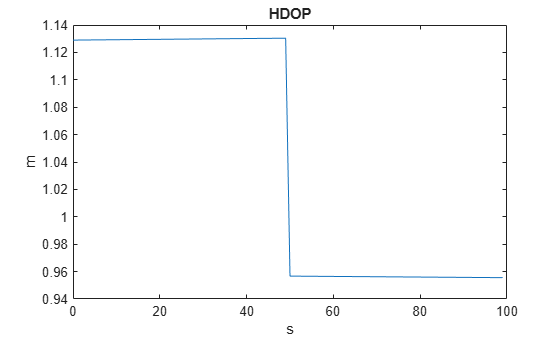Verify that the satellite geometry has changed. Find the index where the HDOP decreased and see if that corresponds to a change in the number of satellites in view. The `numSats` variable increases from 7 to 8.

```% Find expected sample index for a change in the % number of satellites in view. [~, satChangeIdx] = max(abs(diff(hdops))); % Visualize the satellite geometry before the % change in HDOP. satAz = status(satChangeIdx).SatelliteAzimuth; satEl = status(satChangeIdx).SatelliteElevation; numSats = numel(satAz); skyplot(satAz, satEl); title(sprintf('Satellites in View: %d\nHDOP: %.4f', ... numSats, hdops(satChangeIdx)))```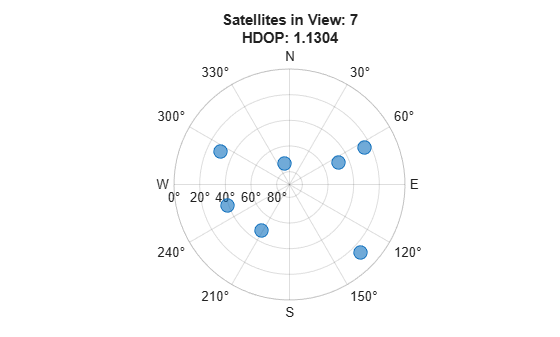```% Visualize the satellite geometry after the % change in HDOP. satAz = status(satChangeIdx+1).SatelliteAzimuth; satEl = status(satChangeIdx+1).SatelliteElevation; numSats = numel(satAz); skyplot(satAz, satEl); title(sprintf('Satellites in View: %d\nHDOP: %.4f', ... numSats, hdops(satChangeIdx+1)))```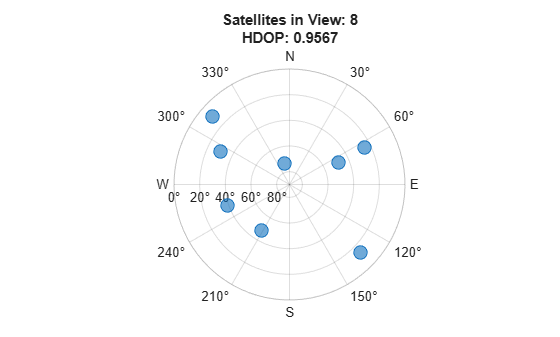The HDOP and VDOP values can be used as diagonal elements in measurement covariance matrices when combining GNSS receiver position estimates with other sensor measurements using a Kalman filter.

```% Convert HDOP and VDOP to a measurement covariance matrix. hdop = status(1).HDOP; vdop = status(1).VDOP; measCov = diag([hdop.^2/2, hdop.^2/2, vdop.^2]); disp(measCov)```
``` 0.6373 0 0 0 0.6373 0 0 0 3.6233 ```